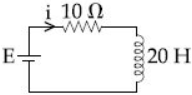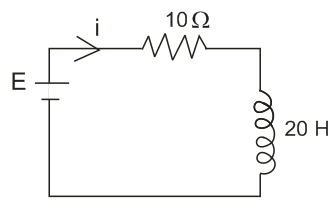# A 20 Henry inductor coil is connected to a 10 ohm resistance in series as shown in figure. The time at which rate of dissipation of energy (Joule's heat) across resistance is equal to the rate at which magnetic energy is stored in the inductor, is :Option 1)Option 2)Option 3)  Option 4)For L-R circuit

where

and rate of magnetic energy stored in inductor =

and rate of dissipiation of energy across Resistance =

from question

So

Option 1)

Option 2)

Option 3)

Option 4)

Exams
Articles
Questions